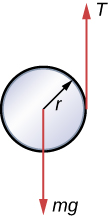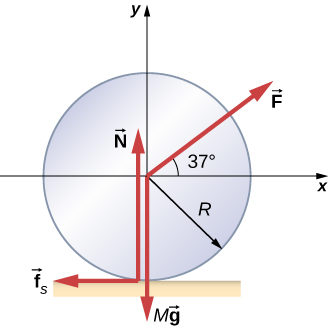# 11.1 Rolling motion  (Page 5/6)

 Page 5 / 6
${v}_{\text{CM}}=\sqrt{\left(3.71\phantom{\rule{0.2em}{0ex}}\text{m}\text{/}{\text{s}}^{2}\right)25.0\phantom{\rule{0.2em}{0ex}}\text{m}}=9.63\phantom{\rule{0.2em}{0ex}}\text{m}\text{/}\text{s}\text{.}$

## Significance

This is a fairly accurate result considering that Mars has very little atmosphere, and the loss of energy due to air resistance would be minimal. The result also assumes that the terrain is smooth, such that the wheel wouldn’t encounter rocks and bumps along the way.

Also, in this example, the kinetic energy, or energy of motion, is equally shared between linear and rotational motion. If we look at the moments of inertia in [link] , we see that the hollow cylinder has the largest moment of inertia for a given radius and mass. If the wheels of the rover were solid and approximated by solid cylinders, for example, there would be more kinetic energy in linear motion than in rotational motion. This would give the wheel a larger linear velocity than the hollow cylinder approximation. Thus, the solid cylinder would reach the bottom of the basin faster than the hollow cylinder.

## Summary

• In rolling motion without slipping, a static friction force is present between the rolling object and the surface. The relations ${v}_{\text{CM}}=R\omega ,{a}_{\text{CM}}=R\alpha ,\phantom{\rule{0.2em}{0ex}}\text{and}\phantom{\rule{0.2em}{0ex}}{d}_{\text{CM}}=R\theta$ all apply, such that the linear velocity, acceleration, and distance of the center of mass are the angular variables multiplied by the radius of the object.
• In rolling motion with slipping, a kinetic friction force arises between the rolling object and the surface. In this case, ${v}_{\text{CM}}\ne R\omega ,{a}_{\text{CM}}\ne R\alpha ,\phantom{\rule{0.2em}{0ex}}\text{and}\phantom{\rule{0.2em}{0ex}}{d}_{\text{CM}}\ne R\theta$ .
• Energy conservation can be used to analyze rolling motion. Energy is conserved in rolling motion without slipping. Energy is not conserved in rolling motion with slipping due to the heat generated by kinetic friction.

## Conceptual questions

Can a round object released from rest at the top of a frictionless incline undergo rolling motion?

No, the static friction force is zero.

A cylindrical can of radius R is rolling across a horizontal surface without slipping. (a) After one complete revolution of the can, what is the distance that its center of mass has moved? (b) Would this distance be greater or smaller if slipping occurred?

A wheel is released from the top on an incline. Is the wheel most likely to slip if the incline is steep or gently sloped?

The wheel is more likely to slip on a steep incline since the coefficient of static friction must increase with the angle to keep rolling motion without slipping.

Which rolls down an inclined plane faster, a hollow cylinder or a solid sphere? Both have the same mass and radius.

A hollow sphere and a hollow cylinder of the same radius and mass roll up an incline without slipping and have the same initial center of mass velocity. Which object reaches a greater height before stopping?

The cylinder reaches a greater height. By [link] , its acceleration in the direction down the incline would be less.

## Problems

What is the angular velocity of a 75.0-cm-diameter tire on an automobile traveling at 90.0 km/h?

${v}_{\text{CM}}=R\omega \phantom{\rule{0.2em}{0ex}}⇒\omega =66.7\phantom{\rule{0.2em}{0ex}}\text{rad/s}$

A boy rides his bicycle 2.00 km. The wheels have radius 30.0 cm. What is the total angle the tires rotate through during his trip?

If the boy on the bicycle in the preceding problem accelerates from rest to a speed of 10.0 m/s in 10.0 s, what is the angular acceleration of the tires?

$\alpha =3.3\phantom{\rule{0.2em}{0ex}}\text{rad}\text{/}{\text{s}}^{2}$

Formula One race cars have 66-cm-diameter tires. If a Formula One averages a speed of 300 km/h during a race, what is the angular displacement in revolutions of the wheels if the race car maintains this speed for 1.5 hours?

A marble rolls down an incline at $30\text{°}$ from rest. (a) What is its acceleration? (b) How far does it go in 3.0 s?

${I}_{\text{CM}}=\frac{2}{5}m{r}^{2},\phantom{\rule{0.2em}{0ex}}{a}_{\text{CM}}=3.5\phantom{\rule{0.2em}{0ex}}\text{m}\text{/}{\text{s}}^{2};\phantom{\rule{0.2em}{0ex}}x=15.75\phantom{\rule{0.2em}{0ex}}\text{m}$

Repeat the preceding problem replacing the marble with a solid cylinder. Explain the new result.

A rigid body with a cylindrical cross-section is released from the top of a $30\text{°}$ incline. It rolls 10.0 m to the bottom in 2.60 s. Find the moment of inertia of the body in terms of its mass m and radius r.

positive is down the incline plane;
${a}_{\text{CM}}=\frac{mg\phantom{\rule{0.2em}{0ex}}\text{sin}\phantom{\rule{0.2em}{0ex}}\theta }{m+\left({I}_{\text{CM}}\text{/}{r}^{2}\right)}⇒{I}_{\text{CM}}={r}^{2}\left[\frac{mg\phantom{\rule{0.2em}{0ex}}\text{sin}30}{{a}_{\text{CM}}}-m\right]$ ,
$x-{x}_{0}={v}_{0}t-\frac{1}{2}{a}_{\text{CM}}{t}^{2}⇒{a}_{\text{CM}}=2.96\phantom{\rule{0.2em}{0ex}}{\text{m/s}}^{2},$
${I}_{\text{CM}}=0.66\phantom{\rule{0.2em}{0ex}}m{r}^{2}$

A yo-yo can be thought of a solid cylinder of mass m and radius r that has a light string wrapped around its circumference (see below). One end of the string is held fixed in space. If the cylinder falls as the string unwinds without slipping, what is the acceleration of the cylinder?A solid cylinder of radius 10.0 cm rolls down an incline with slipping. The angle of the incline is $30\text{°}.$ The coefficient of kinetic friction on the surface is 0.400. What is the angular acceleration of the solid cylinder? What is the linear acceleration?

$\alpha =67.9\phantom{\rule{0.2em}{0ex}}\text{rad}\text{/}{\text{s}}^{2}$ ,
${\left({a}_{\text{CM}}\right)}_{x}=1.5\phantom{\rule{0.2em}{0ex}}\text{m}\text{/}{\text{s}}^{2}$

A bowling ball rolls up a ramp 0.5 m high without slipping to storage. It has an initial velocity of its center of mass of 3.0 m/s. (a) What is its velocity at the top of the ramp? (b) If the ramp is 1 m high does it make it to the top?

A 40.0-kg solid cylinder is rolling across a horizontal surface at a speed of 6.0 m/s. How much work is required to stop it?

$W=-1080.0\phantom{\rule{0.2em}{0ex}}\text{J}$

A 40.0-kg solid sphere is rolling across a horizontal surface with a speed of 6.0 m/s. How much work is required to stop it? Compare results with the preceding problem.

A solid cylinder rolls up an incline at an angle of $20\text{°}.$ If it starts at the bottom with a speed of 10 m/s, how far up the incline does it travel?

Mechanical energy at the bottom equals mechanical energy at the top;
$\frac{1}{2}m{v}_{0}^{2}+\frac{1}{2}\left(\frac{1}{2}m{r}^{2}\right){\left(\frac{{v}_{0}}{r}\right)}^{2}=mgh⇒h=\frac{1}{g}\left(\frac{1}{2}+\frac{1}{4}\right){v}_{0}^{2}$ ,
$h=7.7\phantom{\rule{0.2em}{0ex}}\text{m,}$ so the distance up the incline is $22.5\phantom{\rule{0.2em}{0ex}}\text{m}$ .

A solid cylindrical wheel of mass M and radius R is pulled by a force $\stackrel{\to }{F}$ applied to the center of the wheel at $37\text{°}$ to the horizontal (see the following figure). If the wheel is to roll without slipping, what is the maximum value of $|\stackrel{\to }{F}|?$ The coefficients of static and kinetic friction are ${\mu }_{\text{S}}=0.40\phantom{\rule{0.2em}{0ex}}\text{and}\phantom{\rule{0.2em}{0ex}}{\mu }_{\text{k}}=0.30.$A hollow cylinder is given a velocity of 5.0 m/s and rolls up an incline to a height of 1.0 m. If a hollow sphere of the same mass and radius is given the same initial velocity, how high does it roll up the incline?

Use energy conservation
$\frac{1}{2}m{v}_{0}^{2}+\frac{1}{2}{I}_{\text{Cyl}}{\omega }_{0}^{2}=mg{h}_{\text{Cyl}}$ ,
$\frac{1}{2}m{v}_{0}^{2}+\frac{1}{2}{I}_{\text{Sph}}{\omega }_{0}^{2}=mg{h}_{\text{Sph}}$ .
Subtracting the two equations, eliminating the initial translational energy, we have
$\frac{1}{2}{I}_{\text{Cyl}}{\omega }_{0}^{2}-\frac{1}{2}{I}_{\text{Sph}}{\omega }_{0}^{2}=mg\left({h}_{\text{Cyl}}-{h}_{\text{Sph}}\right)$ ,
$\frac{1}{2}m{r}^{2}{\left(\frac{{v}_{0}}{r}\right)}^{2}-\frac{1}{2}\frac{2}{3}m{r}^{2}{\left(\frac{{v}_{0}}{r}\right)}^{2}=mg\left({h}_{\text{Cyl}}-{h}_{\text{Sph}}\right)$ ,
$\frac{1}{2}{v}_{0}^{2}-\frac{1}{2}\frac{2}{3}{v}_{0}^{2}=g\left({h}_{\text{Cyl}}-{h}_{\text{Sph}}\right)$ ,
${h}_{\text{Cyl}}-{h}_{\text{Sph}}=\frac{1}{g}\left(\frac{1}{2}-\frac{1}{3}\right){v}_{0}^{2}=\frac{1}{9.8\phantom{\rule{0.2em}{0ex}}\text{m}\text{/}{\text{s}}^{2}}\left(\frac{1}{6}\right)\left(5.0\phantom{\rule{0.2em}{0ex}}\text{m}\text{/}{\text{s)}}^{2}=0.43\phantom{\rule{0.2em}{0ex}}\text{m}$ .
Thus, the hollow sphere, with the smaller moment of inertia, rolls up to a lower height of $1.0-0.43=0.57\phantom{\rule{0.2em}{0ex}}\text{m}\text{.}$

#### Questions & Answers

Suppose the master cylinder in a hydraulic system is at a greater height than the cylinder it is controlling. Explain how this will affect the force produced at the cylinder that is being controlled.
Louise Reply
Why is popo less than atmospheric? Why is popo greater than pipi?
Louise
The old rubber boot shown below has two leaks. To what maximum height can the water squirt from Leak 1? How does the velocity of water emerging from Leak 2 differ from that of Leak 1? Explain your responses in terms of energy.
Louise
David rolled down the window on his car while driving on the freeway. An empty plastic bag on the floor promptly flew out the window. Explain why.
Louise Reply
the pressure differential exerted a force on the bag greater than the gravitational force holding it on the floor.
gtitboi
what is angular velocity
Sthandazile Reply
The rate of change in angular displacement is defined as angular velocity.
Manorama
a length of copper wire was measured to be 50m with an uncertainty of 1cm, the thickness of the wire was measured to be 1mm with an uncertainty of 0.01mm, using a micrometer screw gauge, calculate the of copper wire used
Nicole Reply
What is the answer please
Mustapha
If centripetal force is directed towards the center,why do you feel that you're thrown away from the center as a car goes around a curve? Explain
Maira Reply
if there is a centripetal force it means that there's also a centripetal acceleration, getting back to your question, just imagine what happens if you pull out of a car when it's quickly moving or when you try to stop when you are running fast, anyway, we notice that there's always a certain force..
Lindomar
... that tends to fight for its previous direction when you try to attribute to it an opposite one ou try to stop it.The same thing also happens whe a car goes around a curve, the car it self is designed to a"straight line"(look at the position of its tyres, mainly the back side ones), so...
Lindomar
... whenever it goes around a curve, it tends to throw away its the occupiers, it's given to the fact that it must interrupt its initial direction and take a new one.
Lindomar
Which kind of wave does wind form
Matthias Reply
calculate the distance you will travel if you mantain an average speed of 10N m/s for 40 second
Abdulai Reply
400m/s
Feng
hw to calculate the momentum of the 2000.0 elephant change hunter at a speed of 7.50 m/s
Kingsley Reply
how many cm makes 1 inches
Hassan Reply
2.5
omwoyo
2.54cm=1inche
omwoyo
how do we convert from m/s to km/hr
Toni Reply
When paddling a canoe upstream, it is wisest to travel as near to the shore as possible. When canoeing downstream, it may be best to stay near the middle. Explain why?
SANA Reply
Explain why polarization does not occur in sound
Nuradeen
one ship sailing east with a speed of 7.5m/s passes a certain point at 8am and a second ship sailing north at the same speed passed the same point at 9.30am at what distance are they closet together and what is the distance between them then
Kuber Reply
density of a subtance is given as 360g/cm,put it in it s.i unit form
Linda Reply
if m2 is twice of m1. find the ration of kinetic energy in COM system to lab system of elastic collision
Raman Reply
What is a volt equal to?
Clifton Reply

### Read also:

#### Get Jobilize Job Search Mobile App in your pocket Now!

Source:  OpenStax, University physics volume 1. OpenStax CNX. Sep 19, 2016 Download for free at http://cnx.org/content/col12031/1.5
Google Play and the Google Play logo are trademarks of Google Inc.

Notification Switch

Would you like to follow the 'University physics volume 1' conversation and receive update notifications?By OpenStaxBy OpenStaxBy Anh DaoBy OpenStaxBy OpenStaxBy Tony PizurBy RhodesBy OpenStaxBy Brooke DelaneyBy JavaChamp Team